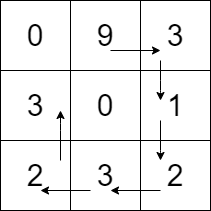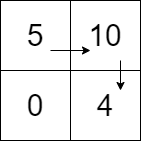1

Path with Maximum Gold

Difficulty: MEDIUM
Avg. time to solve
25 min
Success Rate
75%

Problem Statement

Your task is to return the maximum amount of gold you can collect using the following conditions:

1. Every time you reach a cell, you collect all the gold present in that cell.

2. You can go one step left, right, up, or down from your current cell’s position.

3. You can’t visit the cell which you have already visited.

4. You can’t visit a cell with 0 gold.

5. You can choose any cell to start and stop collecting gold.
Input Format:
The first line of the input contains an integer T denoting the number of test cases.

Each test case’s first line contains two space-separated integers N and M, denoting the rows and columns in the grid respectively.

The next N lines of each test case contain M space-separated integers denoting the amount of gold present in a cell.
Output Format:
For every test case, print a single line containing a single integer denoting the maximum amount of gold you can collect.

The output of each test case will be printed in a separate line.
Note :
You do not need to print anything; it has already been taken care of. Just implement the given function.
Constraints:
1 <= T <= 5
1 <= N, M <= 10
0 <= GRID[i][j] <=  10 ^ 5

Time limit: 1 sec.
1
3 3
0 9 3
3 0 1
2 3 2
23
Explanation of Sample Input 1:
The optimal way to collect maximum gold is 9->3->1->2->3->2->3, or we start from the 2nd element of the first column, i.e. ‘3’ and follow the path 3->2->3->2->1->3->9, either way, we’ll get the same amount of gold.1
2 2
5 10
0 4
19
Explanation of Sample Input 2:
The optimal way to collect maximum gold is 5->10->4 or 4->10->5; either way, we’ll be able to get the same amount of gold.Console